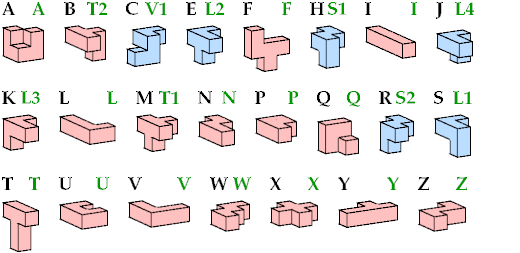# Inflated Pentacubes

A pentacube is a solid made of five cubes joined face to face. There are 29 pentacubes, distinguishing mirror images:The six blue tiles have left- and right-handed forms. Kate Jones's systematic names are shown in green. The mirror forms of V1, S1–S2, and L1–L4 are called V2, N1–N2, and J1–J4. But L3 and J3 are identical because they have mirror symmetry through a plane diagonal.

In 1994 Rodolfo Kurchan published the first issue of Puzzle Fun. It introduced inflated pentominoes, rectangular shapes formed by the 12 pentominoes, where some pentominoes were expanded by a factor of 2 or 3.Here I show all rectangular boxes that can be formed by the 29 pentacubes where one of them is expanded by a factor of 2. Such boxes must have a volume of 180 cells. Click on an entry in the table to see a box tiling with the given format and expanded pentacube.

2×3×30 2×5×18 2×6×15 2×9×10 3×3×20 3×4×15 3×5×12 3×6×10 4×5×9 5×6×6
A                 O O
B                 O O
C                 O O
E                 O O
F     O O       O   O
H                 O O
I O O O O O O O O
J                 O O
K                 O O
L   O O O   O O O O
M                 O O
N   O O O   O O O O
P   O O O   O O O O O
Q                 O O
R                 O O
S                 O O
T     O O       O   O
U   O O O   O O O O O
V     O O       O   O
W     O O       O   O
X     O O       O   O
Y   O O O   O O O O
Z     O O       O   O

Last revised 2021-05-13.

Back to Polyform Tiling < Polyform Curiosities
Col. George Sicherman [ HOME | MAIL ]